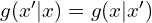# Metropolis采样 （附Python和Matlab代码）时，就回到了Metropolis的方法。

"""
This code is supported by the website: https://www.guanjihuan.com
The newest version of this code is on the web page: https://www.guanjihuan.com/archives/1247
"""

import random
import math
import numpy as np
from scipy.stats import norm
import matplotlib.pyplot as plt

def function(x):  # 期望样品的分布，target distribution function
y = (norm.pdf(x, loc=2, scale=1)+norm.pdf(x, loc=-5, scale=1.5))/2   # loc代表了均值,scale代表标准差
return y

T = 100000  # 取T个样品
pi = [0 for i in range(T)]  # 任意选定一个马尔科夫链初始状态
for t in np.arange(1, T):
pi_star = np.random.uniform(-10, 10)  # a proposed distribution， 例如是均匀分布的，或者是一个依赖pi[t - 1]的分布
alpha = min(1, (function(pi_star) / function(pi[t - 1])))  # 接收率
u = random.uniform(0, 1)
if u < alpha:
pi[t] = pi_star    # 以alpha的概率接收转移
else:
pi[t] = pi[t - 1]  # 不接收转移

pi = np.array(pi)  # 转成numpy格式
print(pi.shape)  # 查看抽样样品的维度
plt.plot(pi, function(pi), '*')  # 画出抽样样品期望的分布   # 或用plt.scatter(pi, function(pi))
plt.hist(pi, bins=100, density=1, facecolor='red', alpha=0.7)   # 画出抽样样品的分布   # bins是分布柱子的个数，density是归一化，后面两个参数是管颜色的
plt.show()

matlab代码的例子：

% This code is supported by the website: https://www.guanjihuan.com
% The newest version of this code is on the web page: https://www.guanjihuan.com/archives/1247

clc;clear all;clf;
s=100000;  % 取的样品数
f=[1,2,3,3,3,3,6,5,4,3,2,1];  % 期望得到样品的分布函数
d=zeros(1,s);  % 初始状态
x=1;
for i=1:s
y=unidrnd(12);  % 1到12随机整数
alpha=min(1,f(y)/f(x)); % 接收率
u=rand;
if u<alpha   % 以alpha的概率接收转移
x=y;
end
d(i)=x;
end
hist(d,1:1:12);

4,757 次浏览

【说明：本站主要是个人的一些笔记和代码分享，内容可能会不定期修改。为了使全网显示的始终是最新版本，这里的文章未经同意请勿转载。引用请注明出处：https://www.guanjihuan.com

（1）在保留浏览器缓存的前提下，目前支持72小时自主修改或删除个人评论。如果自己无法修改或删除评论，可再次评论或联系我。如有发现广告留言，请勿点击链接，博主会不定期删除。
（2）评论支持Latex公式。把latexpage作为标签放在任何位置，评论中的公式可正常编译，示例：
$Latex formula$  [latexpage]